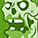# PSoC™ 6 Forum DiscussionsLevel 1
Level 1# How to define project macro in Modus

Dear Community

We are developing 3 Models in 1 product.
for convenience, I want to define a MACRO in the Modus IDE with common code for 3 models.
How to define MACRO in the Modus IDE.

For eg:
If revise the MACRO = MODEL_1,
We can compile the code which is in #if (MODEL == MODEL_1).
If revise the MACRO = MODEL_2,
We can compile the code which is in #if (MODEL == MODEL_2).
If revise the MACRO = MODEL_3,
We can compile the code which is in #if (MODEL == MODEL_3).

Best regards.ModeratorModerator# Re: How to define project macro in Modus

Since you have 3 models you can create a MACRO by defining it by:

#define MODEL "MODEL_1"

If the macro is refined as shown, it will execute the code which is in #if (MODEL == "MODEL_1")

For choosing MODEL_2 you can revise the macro by:

#define MODEL "MODEL_2"

Similarly, you can revise it for "MODEL_3"

Thanks and Regards,
Leo